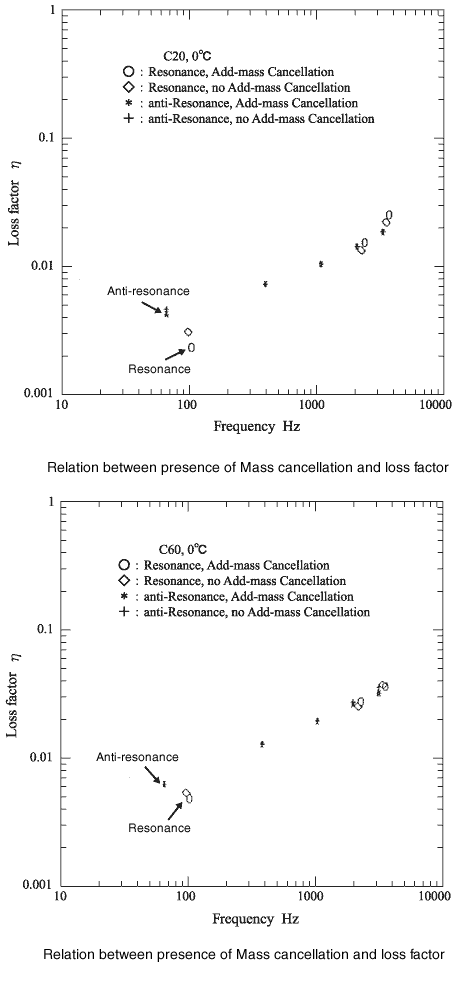Technical Report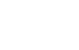Damping materials and their performance measurement
(page5)

13. Selection of methods (Cantilever method and Central exciting method)

Please note that the cantilever method and the central exciting method have the following characteristics in usage:

 Cantilever method Central exciting method Simplicity of testing temperature ○ △ Correspondence to the high temperature × With Vibrochamber Clamp problem Yes No Measurement of the 1st mode Not available Available Measurement of the high times mode difficult Easy Mass cancellation Not required Required Measurement for single soft material Available Not available Measurement at anti-resonance side difficult Easy Price ○ ×

14.Comparisons of loss factors obtained from various methods

Using the specimen data obtained from the cantilever method, the loss factor and Young's modulus of the damping material are calculated in the form of a reduced frequency nomogram. Then, using this nomogram, the loss factor for impedance, SAE, and MIL method is estimated as follow. The relation between the estimated loss factor on vertical axis and the actual value of loss factor calculated by Impedance, SAE, MIL method on horizontal axis is plotted on the following figure. In the result of this figure, the correlation coefficient is 0.946, and the loss factor obtained from Cantilever method corresponds well with the one obtained from Impedance, SAE, MIL method.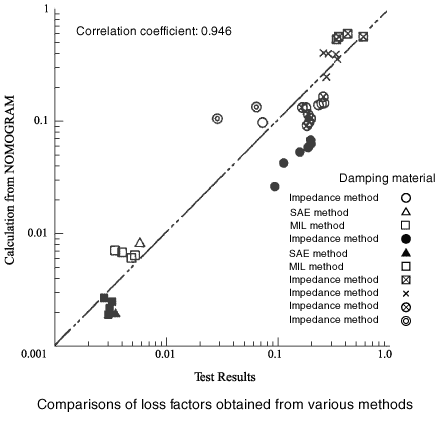15.Mass cancellation

When the central exciting method is performed, generally, the loss factor is measured by measuring the driving point impedance, mobility with an impedance head. In this case, the impedance head itself has a mass for measuring acceleration, even if the force is set as zero, this mass will be actually measured by the force sensor. Hence, the weight of specimen itself and mass are measured, impedance will not be measured properly.  Therefore, it is necessary to correct this additional mass in advance. This operation is called as mass cancellation.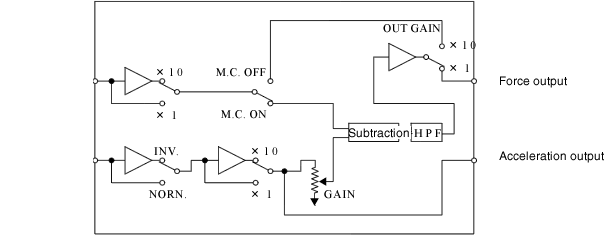The above figure shows one of example of mass cancellation, the figure below shows one of example of test system to conduct mass cancelation.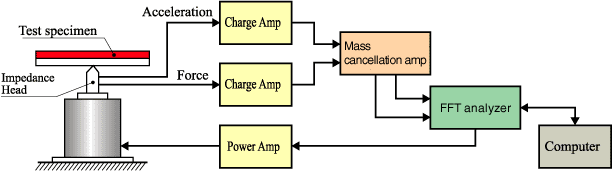The figure below shows one of example of Impedance which is calculated by the above system. It found the resonance frequency different by doing mass cancelation or no mass cancellataion.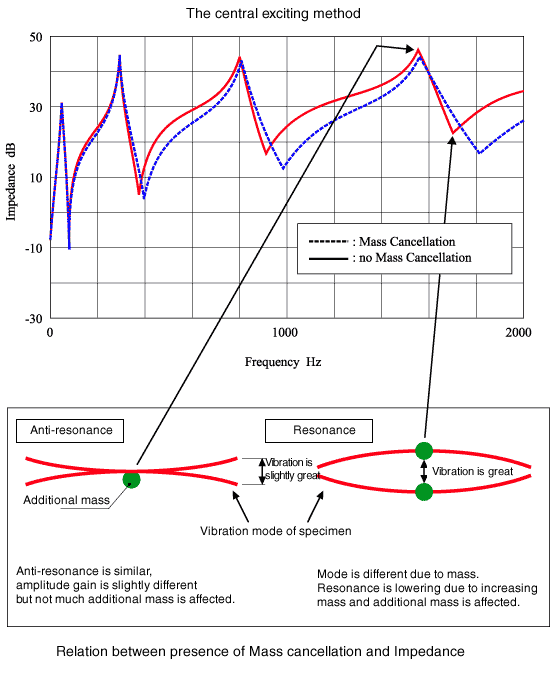Calculation by mass cancelation which is calculated by FFT

Green: Mobility without mass cancelation

Blue: Mobility after doing mass cancelation obtained from calculation 1[(V / F)-(V / F0)]
Red: Mobility after doing mass cancelation with mass cancelation amplifier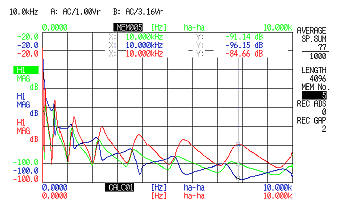Green: Mobility without mass cancelation
Blue: Mobility after doing mass cancelation obtained from calculation 2 [(V / F)(1 / H)-(V / F0)(1 / H)](1 / H)
Red: Mobility after doing mass cancelation with mass cancelation amplifier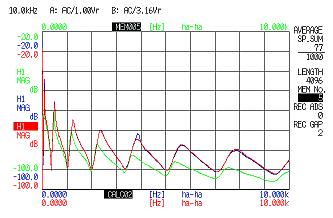In case the mechanical impedance waveform is omitted, but the mass cancellation is performed by FFT, The function of numerator F (mechanical impedance) can be subtracted as it is like(F / V)-( F0 / V).
If the denominator uses a function of F (mobility, etc.), first bring F to the numerator by inverse calculation of the frequency response function (1 / H), subtract the additional mass by the inverse calculation. It is necessary to perform the inverse calculation and return the function that has the original denominator as F. According to the frequency response function without work as FRF1, the frequency response function adding work, no mass cancelation as FRF2, the frequency function after mass cancelation as FRF3, the actual calculation as follow:

FRF3 = 1/((1/FRF2)-(1/FRF1))

The figure below shows the relation between the presence of mass cancelation and loss factor as the central exciting method. When the loss factor is smaller than 0.01, it can be seen that the loss factor is slightly different depending on the presence of mass cancellation.